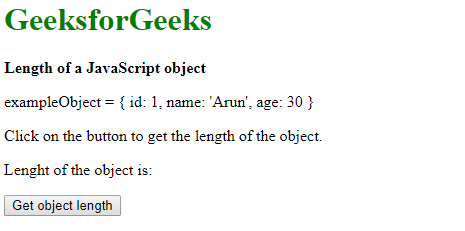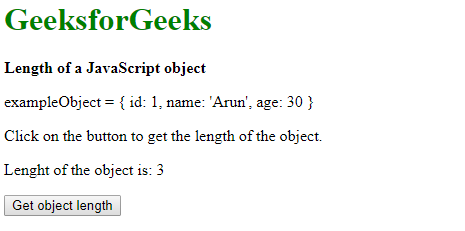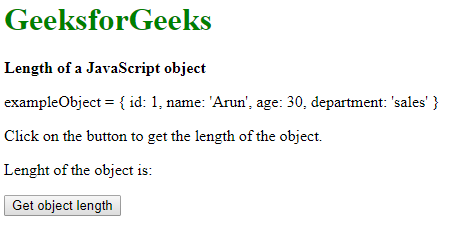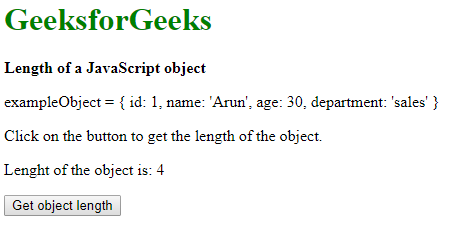# Find the length of a JavaScript object

Method 1: Using the Object.keys() method: The Object.keys() method is used to return the object property name as an array. The length property is used to get the number of keys present in the object. It gives the length of the object.

Syntax:

`objectLength = Object.keys(exampleObject).length`

Example:

 ` ` `<``html``> ` ` `  `<``head``> ` `    ``<``title``>Length of a JavaScript object ` ` ` ` `  `<``body``> ` `    ``<``h1` `style``=``"color: green"``>GeeksforGeeks ` `     `  `    ``<``b``>Length of a JavaScript object ` `     `  `    ``<``p``> ` `        ``exampleObject = { ` `            ``id: 1, ` `            ``name: 'Arun', ` `            ``age: 30 ` `        ``} ` `    `` ` `     `  `    ``<``p``> ` `        ``Click on the button to get ` `        ``the length of the object. ` `    `` ` `     `  `    ``<``p``> ` `        ``Length of the object is:  ` `        ``<``span` `class``=``"output"``> ` `    `` ` ` `  `    ``<``button` `onclick``=``"getObjectLength()"``> ` `        ``Get object length ` `    `` ` `     `  `    ``<``script``> ` `        ``function getObjectLength() { ` `             `  `            ``// Declare an object ` `            ``exampleObject = { ` `                ``id: 1, ` `                ``name: 'Arun', ` `                ``age: 30 ` `            ``} ` ` `  `            ``// Using Object.keys() method to get length ` `            ``objectLenght = Object.keys(exampleObject).length; ` ` `  `            ``document.querySelector('.output').textContent ` `                    ``= objectLenght; ` `        ``} ` `    `` ` ` ` ` `  `                     `

Output:

• Before clicking the button:• After clicking the button:Method 2: Loop through all the fields of the object and check their property: The hasOwnProperty() method is used to return a boolean value indicating whether the object has the specified property as its own property. This method can be used to check if each key is present in the object itself. The contents of the object are looped through and if the key is present, the total count of keys is incremented. This gives the length of the object.

Syntax:

```var key, count = 0;

// Check if every key has its own property
for (key in exampleObject) {
if (exampleObject.hasOwnProperty(key))

// If the key is found, add it to the total length
count++;
}
objectLenght = count;
```

Example:

 ` ` `<``html``> ` ` `  `<``head``> ` `    ``<``title``>Length of a JavaScript object ` ` ` ` `  `<``body``> ` `    ``<``h1` `style``=``"color: green"``>GeeksforGeeks ` `     `  `    ``<``b``>Length of a JavaScript object ` `     `  `    ``<``p``> ` `        ``exampleObject = { ` `            ``id: 1, ` `            ``name: 'Arun', ` `            ``age: 30, ` `            ``department: 'sales' ` `        ``} ` `    `` ` `     `  `    ``<``p``> ` `        ``Click on the button to get ` `        ``the length of the object. ` `    `` ` `     `  `    ``<``p``> ` `        ``Length of the object is: ` `        ``<``span` `class``=``"output"``> ` `    `` ` ` `  `    ``<``button` `onclick``=``"getObjectLength()"``> ` `        ``Get object length ` `    `` ` `     `  `    ``<``script``> ` `        ``function getObjectLength() { ` ` `  `            ``// Declare an object ` `            ``exampleObject = { ` `                ``id: 1, ` `                ``name: 'Arun', ` `                ``age: 30, ` `                ``department: 'sales' ` `            ``} ` ` `  `            ``var key, count = 0; ` ` `  `            ``// Check if every key has its own property ` `            ``for (key in exampleObject) { ` `                ``if (exampleObject.hasOwnProperty(key)) ` ` `  `                    ``// If key is found, add it ` `                    ``// to total length ` `                    ``count++; ` `            ``} ` `            ``objectLenght = count; ` ` `  `            ``document.querySelector('.output').textContent ` `                    ``= objectLenght; ` `        ``} ` `    `` ` ` ` ` `  `                     `

Output:

• Before clicking the button:• After clicking the button:My Personal Notes arrow_drop_upIm listening

If you like GeeksforGeeks and would like to contribute, you can also write an article using contribute.geeksforgeeks.org or mail your article to contribute@geeksforgeeks.org. See your article appearing on the GeeksforGeeks main page and help other Geeks.

Please Improve this article if you find anything incorrect by clicking on the "Improve Article" button below.

Improved By : shubham_singh